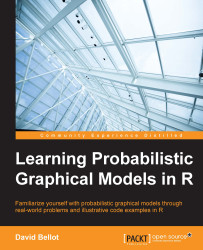•#### Learning Probabilistic Graphical Models in R#### Overview of this book

Probabilistic graphical models (PGM, also known as graphical models) are a marriage between probability theory and graph theory. Generally, PGMs use a graph-based representation. Two branches of graphical representations of distributions are commonly used, namely Bayesian networks and Markov networks. R has many packages to implement graphical models. We’ll start by showing you how to transform a classical statistical model into a modern PGM and then look at how to do exact inference in graphical models. Proceeding, we’ll introduce you to many modern R packages that will help you to perform inference on the models. We will then run a Bayesian linear regression and you’ll see the advantage of going probabilistic when you want to do prediction. Next, you’ll master using R packages and implementing its techniques. Finally, you’ll be presented with machine learning applications that have a direct impact in many fields. Here, we’ll cover clustering and the discovery of hidden information in big data, as well as two important methods, PCA and ICA, to reduce the size of big problems.
Learning Probabilistic Graphical Models in RCreditswww.PacktPub.comPrefaceFree Chapter
Probabilistic ReasoningExact InferenceLearning ParametersBayesian Modeling – Basic ModelsApproximate InferenceBayesian Modeling – Linear ModelsProbabilistic Mixture ModelsAppendixIndex## Machine learning

This book is about a field of science called machine learning, or more generally artificial intelligence. To perform a task, to reach conclusions from data, a computer as well as any living being needs to observe and process information of a diverse nature. For a long time now, we have been designing and inventing algorithms and systems that can solve a problem, very accurately and at incredible speed, but all algorithms are limited to the very specific task they were designed for. On the other hand, living beings in general and human beings (as well as many other animals) exhibit this incredible capacity to adapt and improve using their experience, their errors, and what they observe in the world.

Trying to understand how it is possible to learn from experience and adapt to changing conditions has always been a great topic of science. Since the invention of computers, one of the main goals has been to reproduce this type of skill in a machine.

Machine learning is the study of algorithms that can learn and adapt from data and observation, reason, and perform tasks using learned models and algorithms. As the world we live in is inherently uncertain, in the sense that even the simplest observation such as the color of the sky is impossible to determine absolutely, we needed a theory that can encompass this uncertainty. The most natural one is the theory of probability, which will serve as the mathematical foundation of the present book.

But when the amount of data grows to very large datasets, even the simplest probabilistic tasks can become cumbersome and we need a framework that will allow the easy development of models and algorithms that have the necessary complexity to deal with real-world problems.

By real-world problems, we really think of tasks that a human being is able to do such as understanding people's speech, driving a car, trading the stock exchange, recognizing people's faces on a picture, or making a medical diagnosis.

At the beginning of artificial intelligence, building such models and algorithms was a very complex task and, every time a new algorithm was invented, implemented, and programmed with inherent sources of errors and bias. The framework we present in this book, called probabilistic graphical models, aims at separating the tasks of designing a model and implementing algorithm. Because it is based on probability theory and graph theory, it has very strong mathematical foundations. But also, it is a framework where the practitioner doesn't need to write and rewrite algorithms all the time, for algorithms were designed to solve very generic problems and already exist.

Moreover, probabilistic graphical models are based on machine learning techniques which will help the practitioner to create new models from data in the easiest way.

Algorithms in probabilistic graphical models can learn new models from data and answer all sorts of questions using those data and the models, and of course adapt and improve the models when new data is available.

In this book, we will also see that probabilistic graphical models are a mathematical generalization of many standard and classical models that we all know and that we can reuse, mix, and modify within this framework.

The rest of this chapter will introduce required notions in probabilities and graph theory to help you understand and use probabilistic graphical models in R.

One last note about the title of the book: Learning Probabilistic Graphical Models in R. In fact this title has two meanings: you will learn how to make probabilistic graphical models, and you will learn how the computer can learn probabilistic graphical models. This is machine learning!